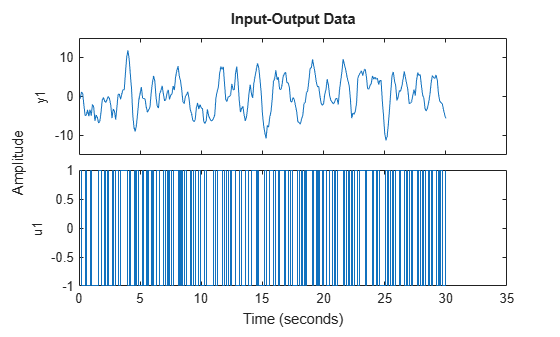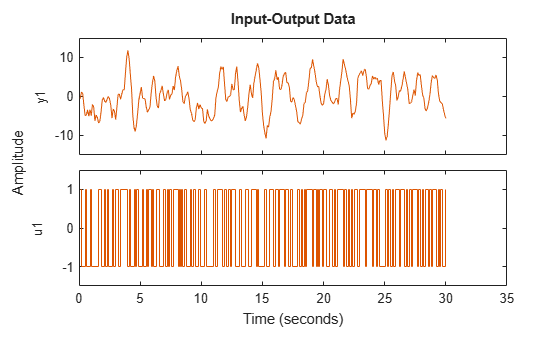## Transform Between Time-Domain and Frequency-Domain Data

System Identification Toolbox™ provides tools for analyzing data and for estimating and evaluating models in both the time and the frequency domains. To use tools and methods that are not in the same domain as your measured data, you can transform your data between the time domain and the frequency domain.

The `iddata` object stores time-domain or frequency-domain data.

• Time-domain data consists of one or more input variables u(t) and one or more output variables y(t), sampled as a function of time.

• Frequency-domain data consists of either transformed input and output time-domain signals or system frequency response sampled as a function of the independent variable frequency.

For detailed information about representing time-domain and frequency-domain data in MATLAB®, see Representing Data in MATLAB Workspace.

You can transform your data from one domain to the other. The table summarizes the commands for transforming data between the time and frequency domains. For more command information, see the corresponding command reference pages.

CommandDescriptionSyntax Example
`fft`

Transform time-domain data to the frequency domain.

You can specify `N`, the number of frequency values.

To transform time-domain `iddata` object `t_data` to frequency-domain `iddata` object `f_data` with `N` frequency points, use:

```f_data = fft(t_data,N)```
`ifft`Transform frequency-domain data to the time domain. Frequencies are linear and equally spaced.

To transform frequency-domain`iddata` object `f_data` to time-domain `iddata` object `t_data`, use:

```t_data = ifft(f_data)```

Converting `iddata` data into the form of an `idfrd` frequency response is a type of estimation. If you want to estimate the frequency response using an `iddata` object, see Transforming Between Frequency-Domain and Frequency-Response Data.

### Transform Data Between Time and Frequency Domains

Transform data from the time domain to the frequency domain and back to the time domain, and compare performance for models estimated from the original and transformed data.

Load and plot the time-domain data `z1`, which contains 300 samples.

```load iddata1 z1 plot(z1)```Find the sample time `Ts` of `z1`.

`Ts = z1.Ts`
```Ts = 0.1000 ```

The sample time is 0.1 s.

Transform `z1` into the frequency domain.

`z1f = fft(z1)`
```z1f = Frequency domain data set with responses at 151 frequencies. Frequency range: 0 to 31.416 rad/seconds Sample time: 0.1 seconds Outputs Unit (if specified) y1 Inputs Unit (if specified) u1 ```

The frequency range extends to 31.416 rad/s, which is equal to the Nyquist frequency of `pi`/`Ts`.

Plot the frequency-domain data.

`plot(z1f)`Transform `z1f` back to the time domain and plot the two time-domain signals together..

`z1t = ifft(z1f)`
```z1t = Time domain data set with 300 samples. Sample time: 0.1 seconds Outputs Unit (if specified) y1 Inputs Unit (if specified) u1 ```
`plot(z1t,z1)`The signals line up precisely.

Estimate second-order state-space models for `z1` and `z1t`.

```sys1 = ssest(z1,2); sys1t = ssest(z1t,2); compare(z1,sys1,sys1t)```Estimate a state-space model for `z1f`.

```sys1f = ssest(z1f,2); compare(z1f,sys1f)```The fit percentages for the time-domain and frequency-domain models are similar.